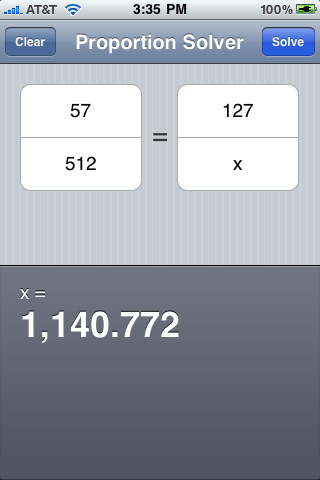Proportion Solver is a simple application that solves proportions. Enter one complete ratio and then a single value for the other ratio, Proportion Solver will solve for the non-entered value in the incomplete ratio.Proportions are useful for comparing ratios (e.g. width/height, Fahrenheit/Celsius, weight/height, mph/meters, U.S. dollars/Euros, pixels/inches, etc.) and are used frequently in high school and college math classes.

http://appshopper.com/utilities/proportion-solver